Lunch

Seven classmates go every day for lunch. If they always come to the front in a different order, will be enough school year to take of all the possibilities?

Result

n =  5040

Solution:Leave us a comment of this math problem and its solution (i.e. if it is still somewhat unclear...):Be the first to comment!To solve this verbal math problem are needed these knowledge from mathematics:

See also our permutations calculator. See also our variations calculator. Would you like to compute count of combinations?

Next similar math problems:

1. Pupils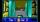Four pupils is going via dark hall. Start at one side and have to get to the other side for 17 minutes. They have only one lamp for the trip. The corridor is narrow, they can therefore go up two pupils at a time and go at the slower speed. Each pupil went.
2. Toys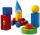3 children pulled 12 different toys from a box. Many ways can be divided toys so that each children had at least one toy?
3. Trainings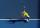The table contains tennis training schedule for Saturday's younger students during the winter indoor season. Before the start of the summer season is preparing a new training schedule. Tomas Kucera will be able to practice only in the morning, sisters Kova
4. NumbersHow many different 7 digit natural numbers in which no digit is repeated, can be composed from digits 0,1,2,3,4,5,6?
5. Olympics metalsIn how many ways can be win six athletes medal positions in the Olympics? Metal color matters.
6. MedalsIn how many ways can be divided gold, silver and bronze medal among 21 contestant?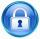Kamila wants to change the password daliborZ by a) two consonants exchanged between themselves, b) changes one little vowel to such same great vowel c) makes this two changes. How many opportunities have a choice?
8. DigitsHow many natural numbers greater than 4000 which are formed from the numbers 0,1,3,7,9 with the figures not repeated, B) How many will the number of natural numbers less than 4000 and the numbers can be repeated?
9. Tricolors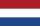From the colors - red, blue, green, black and white, create all possible tricolors.
10. Theorem proveWe want to prove the sentence: If the natural number n is divisible by six, then n is divisible by three. From what assumption we started?
11. Task of the year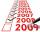Determine the number of integers from 1 to 106 with ending four digits 2006.
12. Election 4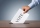In a certain election there are 3 candidates for president 5 for secretory and 2 for tresurer. Find how many ways the election may (turn out/held).
13. DigitsHow many five-digit numbers can be written from numbers 0.3,4, 5, 7 that is divided by 10 and if digits can be repeated.
14. Permutations without repetitionFrom how many elements we can create 720 permutations without repetition?
15. Three-digitHow many three-digit natural numbers do not have the number 7?
16. PIN - codesHow many five-digit PIN - code can we create using the even numbers?
17. VariationsDetermine the number of items when the count of variations of fourth class without repeating is 42 times larger than the count of variations of third class without repetition.